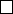5CAT(0) spaces and groups

IV Topics in Geometric Group TheoryTheorem
.
If
X
is a complete, connected length
space of non-positive curvature, then the universal cover
˜
X, equipped with the
induced length metric, is CAT(0).
This was proved by Cartan and Hadamard in the differential geometric
setting.
Corollary.
A (torsion free) group Γ is CAT(0) iff it is the
π
1
of a complete,
connected space X of non-positive curvature.
We’ll indicate some steps in the proof of the theorem.
Lemma.
If
X
is proper, non-positively curved and uniquely geodesic, then
X
is CAT(0).
Proof idea.
The idea is that given a triangle, we cut it up into a lot of small
triangles, and since
X
is locally CAT(0), we can use Alexandrov’s lemma to
conclude that the large triangle is CAT(0).
Recall that geodesics vary continuously with their endpoints. Consider a
triangle ∆ = ∆(
x, y, z
)
¯
B X
, where
¯
B
is a compact ball. By compactness,
there is an ε such that for every x
¯
B, the ball B
x
(¯ε) is CAT(0).
We let
β
t
be the geodesic from
x
to
α
(
t
). Using continuity, we can choose
0 < t
1
< · · · < t
N
= 1 such that
d(β
t
i
(s), β
t
i+1
(s)) < ε
for all s [0, 1].
Now divide ∆ up into a “patchwork” of triangles, each contained in an
ε
ball,
so each satisfies the CAT(0) condition, and apply induction and Alexandrov’s
lemma to conclude.
Now to prove the Cartan–Hadamard theorem, we only have to show that the
universal cover is uniquely geodesic. Here we must use the simply-connectedness
condition.
Theorem.
Let
X
be a proper length space of non-positive curvature, and
p, q X
. Then each homotopy class of paths from
p
to
q
contains a unique
(local) geodesic representative.